## Solution to 1986 Problem 5

 The potential energy of sphere A when it is released is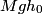$M g h_0$. Therefore its kinetic energy just before it hits sphere B is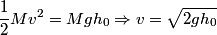\begin{align*}\frac{1}{2} M v^2 = M g h_0 \Rightarrow v = \sqrt{2 g h_0}\end{align*}By conservation of momentum, the velocity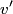$v'$ of the conglomerate just after the collision is\begin{align*}4 M v' = M v \Rightarrow v' = \frac{v}{4} = \frac{\sqrt{2 g h_0}}{4}\end{align*}Therefore, the kinetic energy of the conglomerate is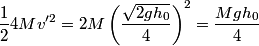\begin{align*}\frac{1}{2}4 M v'^2 = 2 M \left(\frac{\sqrt{2 g h_0}}{4}\right)^2 = \frac{ M g h_0}{4}\end{align*}When the conglomerate reaches its maximum height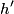$h'$, all of this kinetic energy is converted into potential energy, so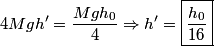\begin{align*}4 M g h' = \frac{ M g h_0}{4} \Rightarrow h' = \boxed{\frac{h_0}{16}}\end{align*}Hence, answer (A) is correct.